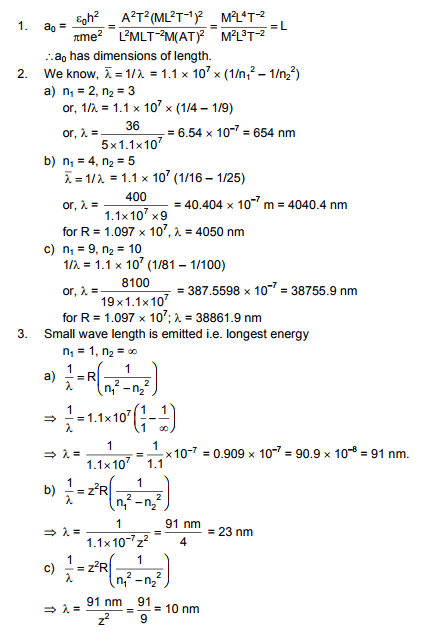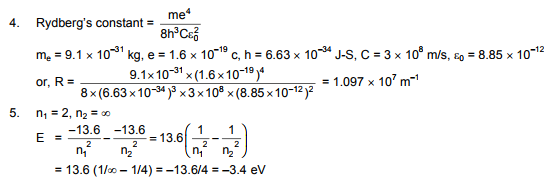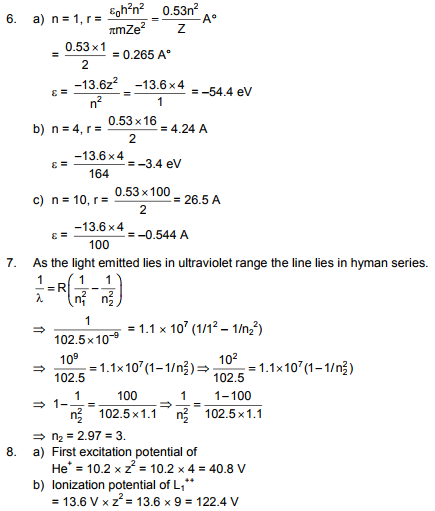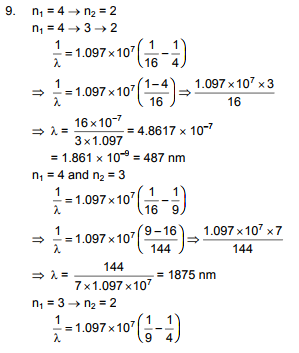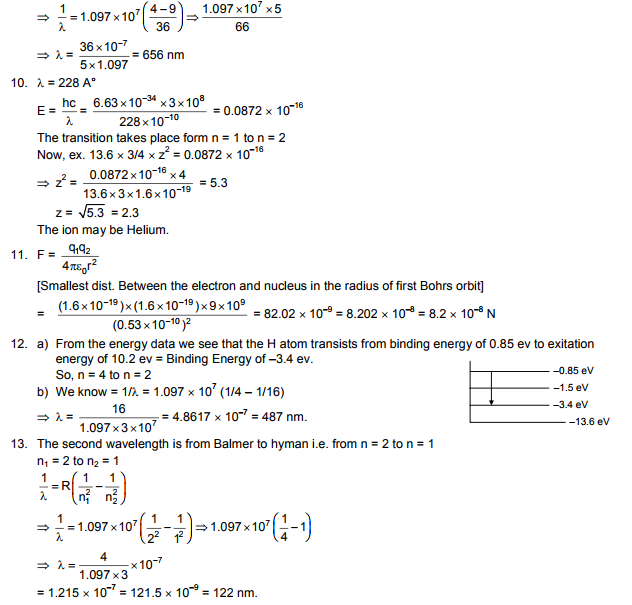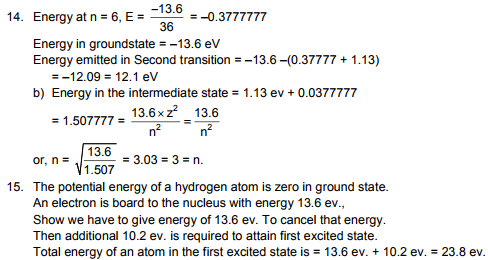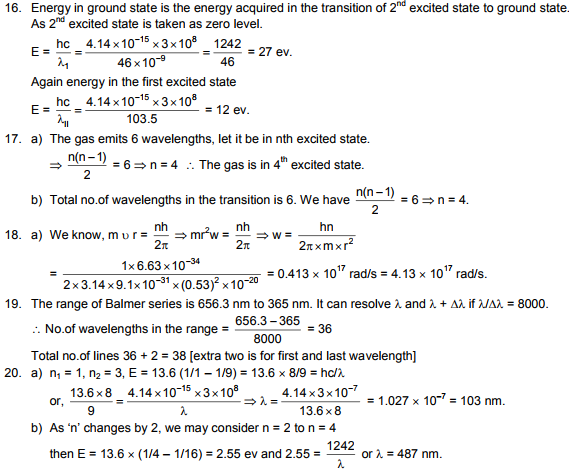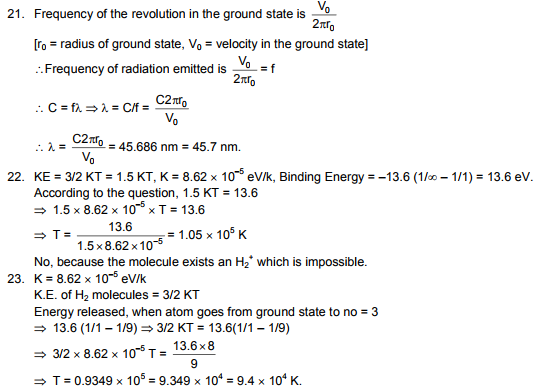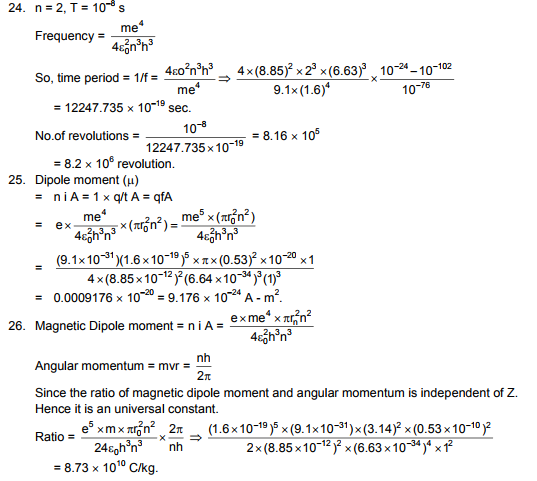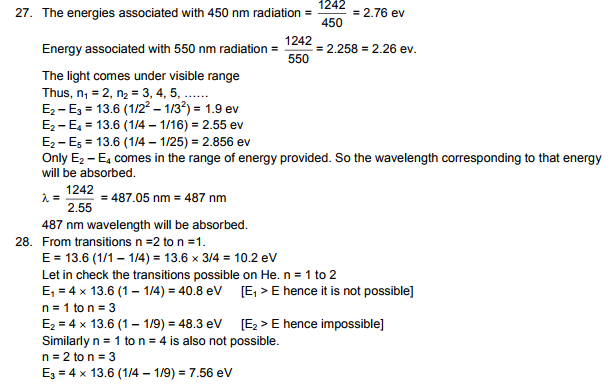# HC Verma Solutions Vol 2 Chapter 21 Bohr’s Theory And Physics Of Atom

HC Verma Solutions Vol 2 Chapter 21 Bohr’s Theory and Physics of Atom gives students the opportunity to learn and solve questions related to the unit in a more effective manner. The solutions offer greater insight into all the exercises and the detailed answers provided in the solutions will further help students to take their preparation to a higher level. The exercises are based on topics such as the dimensional formula of acceleration, wavelength, and radius of atoms etc.  Additionally, some of the problems given include;

• Questions on finding the energy to take out one photon along with finding the intensity and we also have questions on counting the number of photons.
• There are questions related to finding energies associated with types of radiations.
• Questions are also based on Rydberg’s Constant, excitation potential, ionization potential, angular momentum, magnetic dipole moment, etc.
• Finding the frequency of radiation emitted, time period, no. of revolutions, the energy released of atomic particles.

The HC Verma Solutions will prove to useful for Class 12 students who are preparing for competitive exams like JEE. With these solutions, students will also develop better skills to tackle different types of questions and prepare efficiently.

## Key Concepts Related To Bohr’s Theory And Physics Of Atom

Some of the important topics covered in this chapter are;

• Early Atomic Models
• Hydrogen Spectra
• Difficulties With Rutherford’s Model
• The Wave Function of an Electron
• Quantum Mechanics of Hydrogen Atom
• Nomenclature in Atomic Physics
• Laser

## Important Questions In Chapter 21

1. It is said that the numerical value of ionization energy in eV is equal to the ionization potential in volts. If these quantities are measured in some other units what will happen to the equal status?

2. Why is the Balmer series normally observed and analysed before the other series?

3. The numerical value of ionization energy in eV equals the ionization potential in volts. Does the equality hold if these quantities are measured in other units?

4. Which parameters will be the same for all hydrogen-like atoms and ions in their ground states?

(a) the energy of the atom (b) orbital angular momentum of the electron (c) radius of the orbit (d) speed of the electron

5. What happens when a photon stimulates the emission of another photon?

(a) the two photons will have the same wavelength (b) same phase (c) same energy (d) same direction

## HC Verma Solutions Vol 2 Bohr’s Theory And Physics of Atom Chapter 21